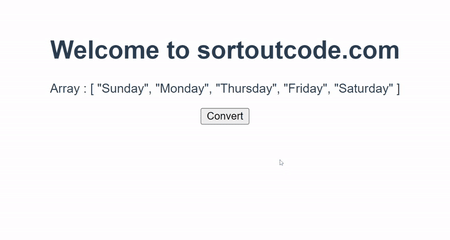# How to convert array into object in VueJS?

Hi Friends 👋,

Welcome To SortoutCode! ❤️

To convert array into object in VueJS. There are several methods to convert array into object, we are going to use `Object.assign()`, `reduce()` and spread oprator `{...[arry]}` methods. And we are going to create custome function to convert the array to object. In these methods `Arry` stand for your array data.

## Short solution

`parentComp.vue`

``````var Arry = [1,2,3,5]; // Array

ArryA = Object.assign({},Arry);
console.log(ArryA); // {0:"a", 1:"b", 2:"c"}

ArryB = {...Arry}
console.log(ArryB); // {0:"a", 1:"b", 2:"c"}

ArryC = ['a', 'b', 'c'].reduce((a, v, i) => ({ ...a, [i]: v}), {})
console.log(ArryC); // {0:"a", 1:"b", 2:"c"}

var ArryD = {};
for (var i = 0; i < Arry.length; ++i)
ArryD[i] = Arry[i];
console.log(ArryD); // {0:"a", 1:"b", 2:"c"}``````

Today, I am going to show you How you convert the array to object in VueJS?

Table of Content

• Setup the Vue (Optional)
• Create FirstComponent.vue and import it into App.js
• Convert an array to object using Object.assign() method
• Convert an array to object using spread oprator
• Convert an array to object using reduce() method
• Convert an array to object using Custome Funtion

This article will guide you to How do I convert array into object in vueJS

## Setup the Vue.js

First, we have to install the Vue project, I had installed the vueJS in my system. If you haven’t installed or have any problem with installation you can follow this article, it will show you step by step process of installation.

How to Install the VueJS project?

## Create FirstComponent.vue and import it into App.js

Create the new component in the `src/components/` components folder. The component name is `FirstComponent.vue` and imported into the `App.js` file:

`App.js`

``````<template>
<FirstComp />
</template>

<script>
import FirstComp from "./components/FirstComp.vue";
export default {
name: "App",
components: {
FirstComp: FirstComp,
},
};
</script>``````

## Convert an array to object using Object.assign() method

To convert array into object in vuejs. We are going to define the array of string `Arry` in the data property. And we are going to convert this array into a object using the `Object.assign({},Arry)`.

Let’s take a short example to better understand.

`FirstComp.vue`

``````<template>
<div class="app-data">
<h1>Welcome to sortoutcode.com</h1>
<div>
<p>Array : {{ Arry }}</p>
<p v-if="output">Output: {{ output }}</p>
<p v-if="output">Type: {{ typeof output }}</p>
<button @click="convertMethodA">Convert</button>
</div>
</div>
</template>
<script>
export default {
name: "FirstComp",
data() {
return {
Arry: ["Sunday", "Monday", "Thursday", "Friday", "Saturday"],
output: "",
};
},
methods: {
convertMethodA() {
this.output = Object.assign({}, this.Arry);
},
},
};
</script>``````

For now, let’s check the output.

## Output## Convert an array to object using spread oprator

To convert array into object in vuejs. We are going to define the array of string `Arry` in the data property. And we are going to convert this array into a object using the spread oprator `{...Arry}` syntex.

Let’s take a short example to better understand.

`FirstComp.vue`

``````<template>
<div class="app-data">
<h1>Welcome to sortoutcode.com</h1>
<div>
<p>Array : {{ Arry }}</p>
<p v-if="output">Output: {{ output }}</p>
<p v-if="output">Type: {{ typeof output }}</p>
<button @click="convertMethodB">Convert</button>
</div>
</div>
</template>
<script>
export default {
name: "FirstComp",
data() {
return {
Arry: ["Sunday", "Monday", "Thursday", "Friday", "Saturday"],
output: "",
};
},
methods: {
convertMethodB() {
this.output = { ...this.Arry };
},
},
};
</script>``````

For now, let’s check the output.

## Output## Convert an array to object using reduce() method

To convert array into object in vuejs. We are going to define the array of string `Arry` in the data property. And we are going to convert this array into a object using the `Arry.reduce()` method.

Let’s take a short example to better understand.

`FirstComp.vue`

``````<template>
<div class="app-data">
<h1>Welcome to sortoutcode.com</h1>
<div>
<p>Array : {{ Arry }}</p>
<p v-if="output">Output: {{ output }}</p>
<p v-if="output">Type: {{ typeof output }}</p>
<button @click="convertMethodA">Convert</button>
</div>
</div>
</template>
<script>
export default {
name: "FirstComp",
data() {
return {
Arry: ["Sunday", "Monday", "Thursday", "Friday", "Saturday"],
output: "",
};
},
methods: {
convertMethodC() {
this.output = this.Arry.reduce((a, v, i) => ({ ...a, [i]: v }), {});
},
},
};
</script>``````

For now, let’s check the output.

## Output## Convert an array to object using Custome Funtion

To convert array into object in vuejs. We are going to define the array of string `Arry` in the data property. And we are going to convert this array into a object using the custome function.

Let’s take a short example to better understand.

`FirstComp.vue`

``````<template>
<div class="app-data">
<h1>Welcome to sortoutcode.com</h1>
<div>
<p>Array : {{ Arry }}</p>
<p v-if="output">Output: {{ output }}</p>
<p v-if="output">Type: {{ typeof output }}</p>
<button @click="convertMethodD">Convert</button>
</div>
</div>
</template>
<script>
export default {
name: "FirstComp",
data() {
return {
Arry: ["Sunday", "Monday", "Thursday", "Friday", "Saturday"],
output: "",
};
},
methods: {
convertMethodD() {
var rv = {};
for (var i = 0; i < this.Arry.length; ++i) rv[i] = this.Arry[i];
this.output = rv;
},
},
};
</script>``````

For now, let’s check the output.

## Output